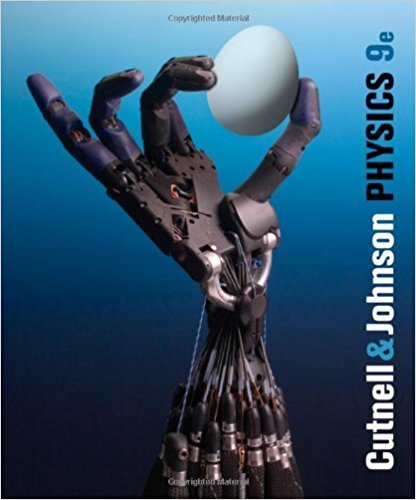×
×

# Solutions for Chapter 14: The Ideal Gas Law and Kinetic Theory## Full solutions for Physics, | 9th Edition

ISBN: 9780470879528Solutions for Chapter 14: The Ideal Gas Law and Kinetic Theory

Solutions for Chapter 14
4 5 0 349 Reviews
15
2
##### ISBN: 9780470879528

This expansive textbook survival guide covers the following chapters and their solutions. This textbook survival guide was created for the textbook: Physics,, edition: 9. Physics, was written by and is associated to the ISBN: 9780470879528. Since 98 problems in chapter 14: The Ideal Gas Law and Kinetic Theory have been answered, more than 73908 students have viewed full step-by-step solutions from this chapter. Chapter 14: The Ideal Gas Law and Kinetic Theory includes 98 full step-by-step solutions.

Key Physics Terms and definitions covered in this textbook
• //

parallel

• any symbol

average (indicated by a bar over a symbol—e.g., v¯ is average velocity)

• °C

Celsius degree

• °F

Fahrenheit degree

×